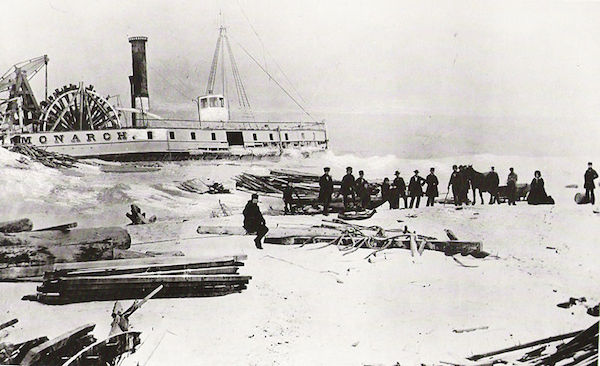Stranded on an Island

Calculus Level 3You are stranded on an island when a boat full of mathematicians floats past. You want to be rescued so you put this integral on a large sign:

$\int_0^{10} {\lfloor x+2 \rfloor}^2 \, dx$

What is the value of this integral?

Bonus: And why were you rescued?

Notation: $\lfloor \cdot \rfloor$ denotes the floor function.

×